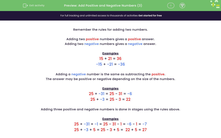# Add Together Three Positive and Negative Numbers

In this worksheet, students will add together three positive and negative numbers.Key stage:  KS 3

Curriculum topic:   Number

Curriculum subtopic:   Use Four Operations for All Numbers

Popular topics:   Subtraction worksheets, Addition worksheets

Difficulty level:#### Worksheet Overview

Remember the rules for adding two numbers.

Examples

15 + 21 = 36

-15 + -21 = -36

Adding a negative number is the same as subtracting the positive.

The answer may be positive or negative depending on the size of the numbers.

Examples

25 + -31 = 25 - 31 = -6

25 + -3 = 25 - 3 = 22

Adding three positive and negative numbers is done in stages using the rules above.

Examples

25 + -31 + -1 = 25 - 31 - 1 = -6 - 1 = -7

25 + -3 + 5 = 25 - 3 + 5 22 + 5 = 27

These are incredibly important rules, to make sure that you remember them!

Let's try some questions now.

### What is EdPlace?

We're your National Curriculum aligned online education content provider helping each child succeed in English, maths and science from year 1 to GCSE. With an EdPlace account you’ll be able to track and measure progress, helping each child achieve their best. We build confidence and attainment by personalising each child’s learning at a level that suits them.

Get started#### Popular Maths topics

••••